GeeksforGeeks App
Open AppBrowser
Continue

# Explore the concept of JavaScript Function Scope and different types of JavaScript Functions

JavaScript is based on functional programming. Therefore, functions are fundamental building blocks of JavaScript. So the function is called first-class citizens in JavaScript.

Syntax:

• Define a function
```function functionName(parameters) {
// SET OF STATEMENTS
} ```
• Call a function
`functionName(arguments); `

The function execution stops when all the statements have been executed or a return statement is found. The return statement stops the execution of the function and returns the value written after the return keyword.

Example: Let’s understand how the function works in javascript

## Javascript

 ``

Output: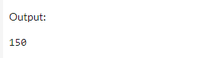Scope: Area of code where that variable is accessible where we can use that variable. It depends where it is defined.

There are basically two scopes:

• Global Scope: When a variable is accessible throughout the program.
• Function Scope: It is also known as Lexical scope. When a variable or function is defined within a particular function then it is accessible only within that function. So its scope is only that function in which variable or function is defined.

1. Variable Scope in function

Example:

## Javascript

 ``

Output: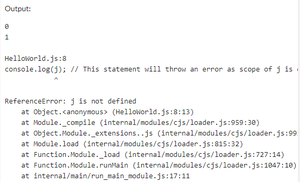Now let’s try to understand the scope of different variables with the same name in different scopes.

Example:

## Javascript

 ``

Output: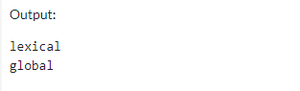So interpreter when reaches to function then it looks for var name is in current scope if it’s not found then it reaches to global scope but here name is defined again inside a function so it gives output lexical and in the outer print, the statement gives output according to the outer scope.

2. Function scope within a function (nested function): The nested function can be called inside the parent function only. The nested function can access the variables of the parent function as well as the global variables. But the parent function cannot access the variables of the inner function. It can be useful when there is a need to create a variable that is passed to function. Using global variables it is not good to create a function because other functions can modify it. so for here, this context nested function is useful. It will prevent the other functions to use this variable. The scope of the nested function is only lexical.

Example: Scope of variables in the nested function.

## Javascript

 ``

Output: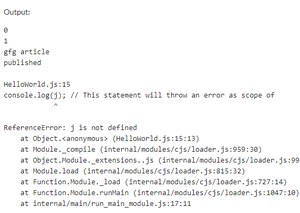Example: In this example, we will create a nested function invocation.

## Javascript

 ``

Output:Here count variable which can only be accessed and modified by the inner function. For scope terms, there are a few terms associated with Execution context and Execution stack

Execution Context: It is something called environment. Every code in javascript has an execution context associated with it in javaScript which means the environment where this code is run. Execution context is created when running that particular piece of code.

Now let’s understand using an example.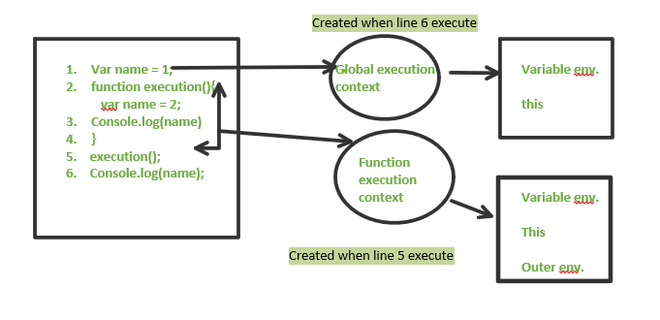Execution context

So here execution context of variable and function is described and within that execution context what parameters are there like for function var, this function, reference to outer execution context is there and this execution context is only created when that function is called or name variable executes to print. so as many times function is called then that many times execution context for that function is created.

Execution stack: It is generally the same as the calling stack used in other programming languages like c, CPP, Java, etc. It creates a stack that maintains a list of all execution context that is currently active. After completion of each execution of that context, it popped from the stack and then the next execution context runs. The global context is the first execution context created each time.

Example: In this example, we will see the working of the Execution stack.

## Javascript

 ``

Output: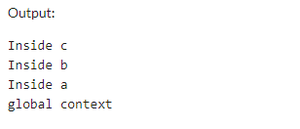Explanation: Here first context is global context then function a call so a context created this an execution context will have information about all the variables that are there in a function and reference to outer context so the outer context for a is global context then next execution context for function b is created will have information about outer context i.e, function a and all the variables inside that so in this way it all works. and after the execution was completed popped out one by one.

Let’s understand how a JavaScript interpreter looks for a variable.

Scope Chain: When a variable is used inside a function, the interpreter looks for that variable inside the function. If the variable is still not found there, then it looks for it in the outer scope i.e. in the parent function. If it is still not found there, it will move to the outer scope of the parent and check it; and do the same until the variable is not found. This search goes on till the global scope and if the variable is not present even in the global scope then the interpreter will throw an error.

Example:

## Javascript

 ``

Output: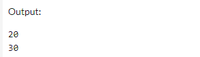Types of Functions in JavaScript: This basically means the way to define a function.

Functions in JavaScript can be defined in two ways:

• Function Definition

Syntax:

```function functionName(parameters) {
// SET OF STATEMENTS
}```
• Function Expression

Syntax:

```var variableName = function (parameters) {
// SET OF STATEMENTS
} ;```

Based on these two different types of functions are there:

Regular Function (Function declaration): Creating a function using function keyword and function name. It has some hoisting properties it holds.

Example: In this example, we will see a Primitive value passed.

## Javascript

 ``

Output:Example: In this example, we will see Non Primitive value passed.

## Javascript

 ``

Output: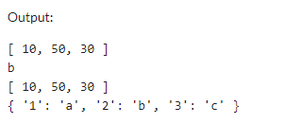Recursive function: calling a function to itself till it reaches base case if base case is not there then stack overflow.

Example:

## Javascript

 ``

Output: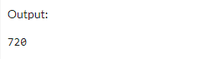Named Function expression – Creating a function as an expression and storing it in a variable. Cannot call a function via function name because it is just a name of the function of the variable so it can only be called via variable name. It resolves the issue of hoisting as for declaration together it needs definition at the same time.

Example:

## Javascript

 ``

Output: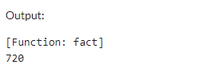Anonymous Function expression: It is the same as function expression just the difference is that not give a name to the function, but the function name is required when the need of stack traces. If the name is not there then the stack takes a variable name as the function name.

Example:

## Javascript

 ``

Output: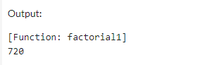Callback function(Function as arguments): Function can be passed as arguments to other functions which means I will be back later.

Two ways:

• Pass function name as an argument to another function
• define the function as an argument to another function

Example:

## Javascript

 ``

Output: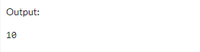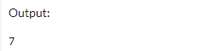Closure Function: The function where an inner function has access to the outer (enclosing) function’s variables—a scope chain (own scope, outer function’s variables, global variables). It is created when an inner function is made accessible from outside of the function that created it means when the outer function returns an inner function. Inner function remembers all of the variables (and their values) that were in scope at the time.

Example:

## Javascript

 ``

Output: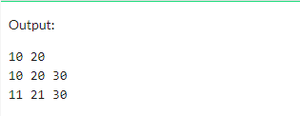Arrow function: It is introduced in Es6 and it is a version of a function expression. It makes it easy to use the function. It has not binding with this function.

Example:

## Javascript

 ``

Output: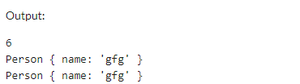7. Constructor Function (Function creates objects): constructor provides a blueprint/structure for objects. The same structure to create multiple objects. Objects can be created by calling the constructor function with the ‘new’ keyword.

Example:

## Javascript

 ``

Output: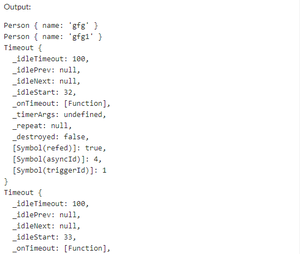My Personal Notes arrow_drop_up
Related Tutorials